Reach Us+44-1474-556909
Single Reverse and Unsymmetrical Vertical Curve for Highways Utilizing Quintic Polynomial Equation of Odd Powers | OMICS International
Journal of Applied & Computational Mathematics
All submissions of the EM system will be redirected to Online Manuscript Submission System. Authors are requested to submit articles directly to Online Manuscript Submission System of respective journal.

Single Reverse and Unsymmetrical Vertical Curve for Highways Utilizing Quintic Polynomial Equation of Odd Powers

Shebl SA*

Engineering Mathematics and Physics Department, Alexandria University, Alexandria, Egypt

*Corresponding Author:
Shebl SA
Faculty of Engineering
EngineeringMathematics and Physics Department
Alexandria University, Alexandria, 2438,Egypt
Tel: +2035910052
E-mail: [email protected]

Received Date:September 01, 2015; Accepted Date: September 21, 2015; Published Date:September 25, 2015

Citation: Shebl SA (2015) Single Reverse and Unsymmetrical Vertical Curve forHighways Utilizing Quintic Polynomial Equation of Odd Powers. J Appl ComputatMath 4:257. doi:10.4172/2168-9679.1000257

Copyright: © 2015 Shebl SA. This is an open-access article distributed under theterms of the Creative Commons Attribution License, which permits unrestricteduse, distribution, and reproduction in any medium, provided the original author andsource are credited.

Visit for more related articles at Journal of Applied & Computational Mathematics

Abstract

The main goals of reasonable geometric design of

unsymmetrical

vertical highway curves are the fulfillment of the two main aspects: sufficient value of the sight distance and avoidance of the sudden change in vertical acceleration, i.e. rider's comfort. Existing asymmetrical vertical highway curves consist of either one or two or even three curves takes the form of parabolic or cubic equations. In the favor of maintaining more sufficient sight distance and curve smoothness, we introduce a new single reverse and unsymmetrical vertical highway curve employing a quintic

polynomial

equation of odd powers. Equation parameters were determined exploiting the given beginning and end grades, and elevations of the points of vertical curvature and vertical tangency. The comparative study presented showed increment ranges between the values of 6.1% to 20.8% of the sight distance. The proposed curve proves smoothness particularly at the beginning of the curve, i.e. improvement of the rider’s comfort along the range of length up to 200 m and greater than 650 m along the curve. Finally, the study demonstrated the suitability of using the curve for different values of beginning and end grades which is impossible to be connected using the other existing curves. Geometric properties and relationships of the curve are presented and justified numerically.

Keywords

Quintic; Unsymmetrical; Vertical curve; Sight distance; Rider comfort

Introduction

Parabolic curves are traditionally used in highway vertical alignment design because their properties make the analysis of curve characteristics easier. For example, a parabola has a constant rate of change in grade that makes the calculation of curve offsets and elevations easier. This feature was useful many years ago, when the calculations were performed manually. With computers, however, the extra mathematical calculations exhibited by the new curve are not an issue.

Existing asymmetrical vertical highway curves consist of either one or two or even three curves takes the form of parabolic or cubic equations for the purposes of accomplishing sufficient value of the sight distance and avoidance of the sudden change in vertical acceleration, i.e. rider's comfort [1,2].

The significance of unsymmetrical vertical curve arose because it enhances the driving comfort, improves the available sight distance and provides larger clearance.

The design guides of the AASHTO suggest that where there are requirements for vertical clearance or other geometric constraints, the unsymmetrical vertical curve is recommended to use .

A cubic parabolic single-arc unsymmetrical vertical curve was introduced by Sun and Chen aiming for the purpose of obtaining the curve minimum length putting into consideration three main limiting factors: driving comfort, least traveling time and the sight distance. The analysis of such curve is limited to the crest vertical curve only .

Easa presented a new and improved unsymmetrical vertical curve for highways that takes the form of a cubic, instead of the traditional parabolic, function . The new curve has a rate of change in grade that gradually varies between PVC and PVT, thus eliminating the sudden change in curvature at the PCC of existing two-arc unsymmetrical curves. As a result, the new curve smoothes out driver comfort along the curve, replacing the sudden change that occurs at the PCC of existing curves. The new curve was developed considering a crest vertical curve, but the results are also applicable to sag vertical curves. The new curve consists of a single arc, unlike existing unsymmetrical vertical curves that consist of two parabolic arcs connected at the point of common curvature. The new curve slightly improved the highway sight distance in most cases.

For constrained vertical alignments, where the beginning and end of a vertical curve are fixed, existing symmetrical and unsymmetrical vertical curves may not satisfy vertical clearance requirements . Accordingly, Easa presented a new three-arc vertical curve which accommodated the vertical clearance and the fixation of the beginning and end of the vertical arc. The curve connects two tangents with unequal lengths and consists of three parabolic arcs smoothly connected at the points of common curvature. The curve improved the sight distance for certain conditions .

Also, Easa in his study introduce a research in which the symmetrical curve was replaced with two arcs, which have two different rates of change of grade and a common point of tangency. The result curve, called the equal-arc unsymmetrical (EAU) curve enhanced sight distance, highway aesthetics, driver comfort, and vertical clearance .

Easa and Hassan used the cubic polynomial to develop a vertical transition curve before and after the parabolic vertical curve. The resulting curve, called transitioned vertical curve, consists of transitionparabolic- transition segments. The minimum length of a transition curve is derived based on the criterion of driver comfort. The new transitioned vertical curve, which exhibits striking similarities to the spiraled horizontal curve .

In this paper, a new single reverse and unsymmetrical vertical curve for highways has been originated. The curve takes the form of a quintic polynomial equation of odd powers, whose parameters may be determined utilizing the values of grades of the beginning and end of curve and elevations of the points of vertical curvature and vertical tangency.

Such curve provides three main advantageous features which convincingly should enhance the design of highway vertical alignments. The first feature is the increasing of the value of the sight distance in comparative to the existing vertical curves. The offered comparative study demonstrates an increment ranges between the values of 6.1% to 20.8% of the sight distance.

The second feature is the smoothness of the curve particularly at the beginning of the curve at which, the rate of changes in slope equals zero and increase gradually. Such smoothness reflected on the avoidance in the sudden change in vertical acceleration at point of vertical curvature; point of common curvature and at point of vertical tangency, which, in turns, the improvement of the rider’s comfort on the highway. The third property is reversal of the curve which effectively enables and facilitates the suitability of using the curve for different values of beginning and end grades which is impossible to be connected using the other existing curves.

Such advantages are investigated numerically and compared with previous cases. Geometric properties of the curve are handled and analyzed for the purpose of field planning and alignment. These include rate of change of slope, highest point, basic and reverse offsets, forward and backward offsets, and sight distance. A notable increase in the sight distance is attained using the curve.

Also, the study of the rider's comfort is analyzed via computing the vertical acceleration along different curve lengths. An improvement in the rider comfort is achieved along certain range along the curve.

Notations

The following are the elements to be used in analyzing the topic:

L: Length of the curve

L1: Length of the first tangent

L2: Length of the second tangent

g1: Slope of the first tangent

g2: Slope of the second tangent

PVI: Point of vertical intersection

PVC: Point of vertical curvature

PVT: Point of Vertical Tangency

PR: Point of Reflection

PCC: Point of Common Curvature

hPVC: Elevation of PVC

hPVT :Elevation of PVT

xht: Highest point

Methodology

Highway vertical alignments consist of straight roadway sections (grades or tangents) connected by vertical parabolic curves. The vertical curves may be symmetrical, unsymmetrical, or reverse. The symmetrical vertical curve is a parabolic arc that connects two tangents such that their horizontal projections are equal. The unsymmetrical (compound) vertical curve consists of two parabolic arcs that connect two tangents with unequal horizontal projections .

There are different types of unsymmetrical vertical curves.

Colcord developed an unsymmetrical curve in which the point of common curvature (PCC) lies at any intermediate point of the vertical curve .

In the traditional unsymmetrical curve, PCC lies under the point of vertical intersection [10,11].

Easa developed an unsymmetrical curve called an equal-arc unsymmetrical curve (EAU) in which PCC lies at the middle of the curve .

Existing symmetrical and unsymmetrical (two curves) vertical curves may not be feasible when the highway alignment is subject to physical constraints (e.g., vertical clearance) and the beginning and end of the vertical curve are fixed.

Curve Proposed Equation

According to Figure 1, we introduce the following items:

L=L1 + L2             (1)

A=g2 - g1             (2)

R=L1 /L             (3)

Equation of the curve may be proposed as follows:

y=a + bx + cx3 + dx5            (4)

hPVT=hPVC + g1 L1 + g2 L2           (5)

where a; b; c and d are the parameters which will be determined according to the highway constraints, i. e. highway spatial features, as follows:

At x=0, y=hPVC, then "(4)" gives:

a=hPVC           (6)

Also, at x=L, y=hPVT

then, "(4)" gives:

hPVT=hPVC + bL + cL3 + dL5           (7)

Since the slope of the curve at any point (x, y) is given by the derivation of y w. r. to x, hence, "4" produces:

dy/dx=b + 3cx2 + 5dx4          (8)

and, at x=0, dy/dx=g1, then "(8)" yields:

b=g1          (9)

and finally, at x=L, dy/dx=g2, then "(8)" yields:

g2=g1 + 3cL2 + 5dL4           (10)

Solving "(7)" and "(10)", then:

c= (A/2L2)(4-5R)           (11)

d=(-A/2L4)(2-3R)           (12)

Thus, equation of the proposed curve associated with the highway spatial features takes the form:

y=hPVC + g1 x + (A/2L2)(4-5R)x3 + (-A/2L4)(2-3R)x5

Curve Geometrical Properties

Geometrical properties of the new reverse and unsymmetrical vertical curve are significant for the purpose of studying and analyzing the structural design of the highway as well as safety and controlled speed considerations. Geometrical planning, design and alignment depend on such geometrical properties as well.

Rate of change of slope

The rate of change in slope of the curve is defined as the second derivation of the equation of the curve w. r. to x, i. e., "(4)" will be:

r= d2y/dx2=6cx + 20dx3           (13)

or, using values of c and d from "(11)" and "(12)", then:

r=(Ax/L4)[3(4-5R)L2 – 10(2-3R)x2]           (14)

or:

r=(Ax/L4)(2-3R)[3L2R1 – 10x2]

Since rate of change in slope equals zero at PVC and at PR, i. e. at x=0 and x=xPR, then "(14)" yields:

xPVC=0          (15)

and

xPR=(-3c/10d)1/2,

or:

xPR=[(3/10)L2 R1] ½           (16)

where:

R1=(4-5R)/ (2-3R)           (17)

At x=L, the rate of change rPVT is:

rPVT=(A/L)(15R-8)          (18)

The minimum rate of slope occurs at distance xmin, Figure 2, and equals rmin, where:

xmin=[(L2/10) R1]1/2          (19)

xmin=(2A/L)(4-5R)(R1/10)1/2           (20)

State of reflexivity

As derived above, location of reverse point PR is located according to the relation:

xPR2=(3/10)L2 R1

from which, we conclude that the curve will not be reversed unless the following condition must be satisfied:

(4/5) L ≤ L1 < (2/3)L           (21)

Highest point

At highest point, the first derivative of equation of the curve, "(8)", equals zero, i.e.:

dy/dx=b + 3cx2 + 5dx4=0,           (22)

from which, the highest point lies at a distance xht from PVC, which is: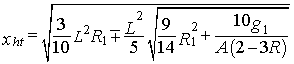(23)

and the elevation of the highest point yht is determined from equation of the curve, "(4)", and equals: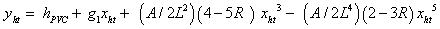(24)

Basic offset

For point A, and from the geometry of Figure 3, the basic offset fx equals: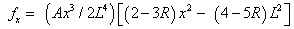(25)

For PVI, where x=L1, the basic offset equals: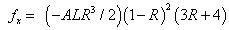(26)

and for PVT, where x=L, the basic offset equals:

fx=(-AL)(1-R)          (27)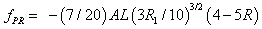(28)

For reverse point PR, the basic offset fPR is:

Reverse offset

Focusing on Figure 4, the reverse offset fr at point A of coordinates (x, y) equals: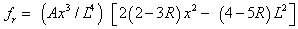(29)

For PVT, where x=L, then the reverse offset equals: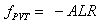(30)

and for reflection point PR, the reverse offset equals: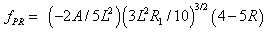(31)

Basic offset fx equals reverse offset fr at: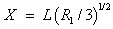Difference between reverse and basic offsets

Difference between reverse and basic offsets may be obtained using "(25)" and "(29)" as: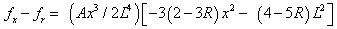For PVT, where x=L, then the difference between reverse and basic offset equals: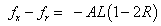(32)

For reflection Point, where x=xPR, then the difference between reverse and basic offset equals: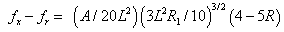(33)

Such difference is represented in Figure 5.

Forward and backward offsets

Referring to the geometry of Figure 6, forward and backward offsets fb and ff may be derived utilizing "25" in terms of distance u before and after given point A of distance x from PVC are: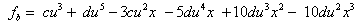(34)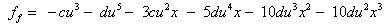(35)

These relations are represented in Figure 7.

And the difference between fb and ff is: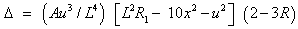(36)

fb and ff are equal at: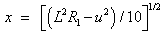(37)

and,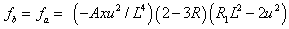at reflection point: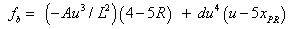(38)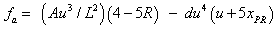(39)

And finally, difference between fb and ff at reflection point is: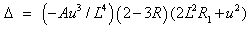(40)

Sight Distance

Sight distance is a fundamental criterion in the design of any highway. It is essential for the driver to be able to perceive hazards on the road, with sufficient time in hand to initiate any required action safely. On a two-lane two-way road it is also necessary for him or her to be able to enter the opposing lane safely while overtaking.

When the sight distance S is less than the length of the curve L the minimum sight distance occurs when the driver (or object) is at PVC and the object (or driver) is on the curve. Let h1=driver’s eye height, h2=object height, and x1 and x2 are distances from the tangent point to the driver and object, respectively.

From Figure 8, employing "25":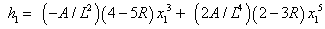(41)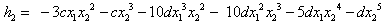(42)

Solving "(41)" and "(42)", the minimum sight distance for the curve, SMn is: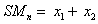(43)

For EAU curve: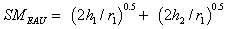(44)

Rider's Comfort

The vertical acceleration on a vertical curve is a measure of rider comfort. As acceleration increases, comfort decreases.

This acceleration is given by :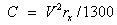(45)

where C is the centrifugal vertical acceleration (m/s2), V is the design speed (km/h), and r is the rate of change in grade (percent change of the grade per m or A/L). For the proposed curve, based on "(13) and "(14)", the rate of change in grade at a distance x from VPC, rx, is given by: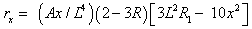hence, acceleration C is: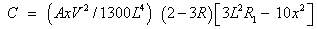(46)

Such relation is represented in Figure 9.

Riding comfort on sag curves typically doesn’t become a problem as long as centripetal acceleration does not exceed 0.3 m/s2 .

With this limit, the relationship between L and A becomes: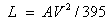(46)

Lengths needed to provide ride comfort are typically about half that needed to meet headlight sight distance requirements, thus headlight, and subsequently stopping, sight distance control the length of a sag curve .

Numerical Justification

According to the highway design associations considerations, including AASHTO , there are critical issues should be fulfilled when acknowledging proposed geometrical design of vertical curve. These are sight distance; rider's comfort and validity of the curve to the various beginning and ending slopes.

Verification of validity of the proposed curve may be established and judged via comparing the above mentioned issues with those related to previous curves.

Sight distance improvement

Consider passing sight distance, where according to AASHTO , the driver eye height (h1) and object height (h2) used in design are equal to 1.08 m. Assuming that g1=0.02, and g2=-0.03, the minimum sight distance was calculated for both the new proposed curve; the "New and improved single-arc unsymmetrical vertical curve for highways" and "the equal-arc unsymmetrical (EAU) vertical curve" developed by Easa  curves for different curve characteristics, as shown in Table 1.

Case L1 L2 Minimum sight distance, SM (m) Increase in SM% Increase in SM%
Proposed Easa EAU
1 320 500 343.9 317.6 313.8 7.6 8.8
2 400 500 383.1 359.9 356.7 6.1 6.9
3 320 600 358.1 315 314.4 12 12.2
4 400 600 394 352.7 351.3 10.5 10.8
5 500 600 440.2 402.5 401.1 8.6 8.9
6 500 1000 486.5 385.5 394.4 20.8 18.9

Table 1: Comparison of minimum sight distance of new proposed; Easa, and EAU curves (h1=h2=1.08 m; g1=0.02, and g2=-0.03).<//

As noted, the new curve provides greater minimum sight distance in all cases. Note that if h1 and h2 are not equal, the minimum sight distance occurs when the shorter height is placed at PVC.

The results were obtained using "(4)"; "(42)", and "(43)", x1 and x2 were calculated Maple software.

The results indicate notable increase in the value of sight distance ranges from 6.1% to 20.8%.

Rider's comfort improvement

Addressing "(46)" and substituting the values of parameters of the new proposed curve calculated for the sight distance calculations, the rider's comfort of the proposed new curve was represented in Figure 1, in comparative to those related to Easa and EAU curves .

As mentioned above, the vertical acceleration on a vertical curve is a measure of rider comfort. As acceleration increases, comfort decreases.

For vertical curves of length L up to 200 m and L greater than 650 m, the new proposed curve has a vertical acceleration less than those of the other two curves, consequently, emitting more comfortable within the stated length (Figure 10).

Figure 10: Comparison between vertical accelerations of the new proposed curve and both Easa and EAU curves.

For curves of length L ranges between 200 m to 650 m, the proposed curve has a vertical acceleration greater than those of the other two curves consequently the curve is less comfortable within the stated length.

Validity of the curve to the various beginning and ending slopes

The following Figures 11-14 give an interesting and significant geometrical property which is the suitability of the unsymmetrical reverse curve for the all combinations of beginning and ending grades. Such figures were graphed using equation of the curve, "(4)".

Conclusion

A new vertical unsymmetrical and reversed vertical highway curve was originated in this paper utilizing quintic polynomial equation of odd powers. Such equation has been formulated in terms of the spatial features of the highway, i.e. the values of grades of the beginning and end of curve and elevations of the points of vertical curvature and vertical tangency.

The geometric properties of the curve has been established and special attention has been paid for the characteristic features of the curve which are the sight distance; rider's comfort and validity of the curve to the various beginning and ending slopes.

Numerical justification has been arranged and has proven a notable beneficial enhancement in such features which recommend the use of such curve for the geometrical design of highways.

A noticeable increase in the value of sight distance for the curve ranges from 6.1% to 20.8% compared to the other common used curve.

The justification showed an approved degree of rider's comfort and also validity of applying the proposed curve for all the possible combinations of beginning and ending grades which requires a compound vertical curve in other geometrical designs.

References

Select your language of interest to view the total content in your interested language

Article Usage

• Total views: 20495
• [From(publication date):
October-2015 - Jun 27, 2019]
• Breakdown by view type
• HTML page views : 16484Can't read the image? click here to refresh